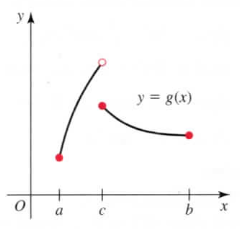×
Get Full Access to Calculus: Early Transcendentals - 1 Edition - Chapter 4.1 - Problem 13e
Get Full Access to Calculus: Early Transcendentals - 1 Edition - Chapter 4.1 - Problem 13e

×

# Solved: Absolute maximum/minimum values from graphs UseISBN: 9780321570567 2

## Solution for problem 13E Chapter 4.1

Calculus: Early Transcendentals | 1st Edition

• Textbook Solutions
• 2901 Step-by-step solutions solved by professors and subject experts
• Get 24/7 help from StudySoup virtual teaching assistantsCalculus: Early Transcendentals | 1st Edition

4 5 1 422 Reviews
14
5
Problem 13E

11-14. Absolute maximum/minimum values from graphs Use the following graphs to identify the points (if any) on the interval [a, b] at which the function has an absolute maximum value or an absolute minimum value.Step-by-Step Solution:
Step 1 of 3

STEP_BY_STEP SOLUTION Step_1 When an output value of a function is a maximum or a minimum over the entire domain of the function, the value is called the absolute maximum or the absolute minimum. Let f be a function with domain D and let c be a fixed c onstant in D. Then the o utput value ) is the 1. Absolute maximum value of f on D if and only if f(x) f(c) , for all x in D. 2. Absolute minimum value of f on D if and only if f(c) f(x) , for all x in D. Step-2 The given graph is ; Now we need to verify the points , from the graph on the interval [a,b] at which the function has an absolute maximum value or an absolute minimum value. From the graph it is clear that the given function y = f(x) is not continuous function on the given interval [a,b].Because the graph has a break at x=c , here c is the interior point of [a,b]. So, the function is not continuous on [a,b]. Observe the graph the given interval is not a closed interval , at x=c the function has a break. So , from the graph it is clear that the function y = f(x) is not in closed interval. so , no absolute maximum value and no absolute minimum value (here the maximum and minimum values are not mentioned).Because the existence of extreme values depend on the both the function and the interval , so the function is not in the closed interval then the function may not attains the absolute extreme values.

Step 2 of 3

Step 3 of 3

##### ISBN: 9780321570567

The full step-by-step solution to problem: 13E from chapter: 4.1 was answered by , our top Calculus solution expert on 03/03/17, 03:45PM. The answer to “?11-14. Absolute maximum/minimum values from graphs Use the following graphs to identify the points (if any) on the interval [a, b] at which the function has an absolute maximum value or an absolute minimum value.” is broken down into a number of easy to follow steps, and 35 words. This full solution covers the following key subjects: absolute, maximum, minimum, Graphs, interval. This expansive textbook survival guide covers 112 chapters, and 7700 solutions. Since the solution to 13E from 4.1 chapter was answered, more than 444 students have viewed the full step-by-step answer. This textbook survival guide was created for the textbook: Calculus: Early Transcendentals, edition: 1. Calculus: Early Transcendentals was written by and is associated to the ISBN: 9780321570567.

## Discover and learn what students are asking

Calculus: Early Transcendental Functions : Product and Quotient Rules and Higher-Order Derivatives
?Using the Product Rule In Exercises 1–6, use the Product Rule to find the derivative of the function. $$g(s)=\sqrt{s}\left(s^{2}+8\right)$$

Calculus: Early Transcendental Functions : The Natural Logarithmic Function: Integration
?In Exercises 1-26, find the indefinite integral. $$\int \frac{x^{2}}{5-x^{3}} d x$$

Unlock Textbook Solution